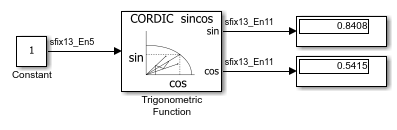Main Content

# sincos Function with Fixed-Point Input

This example shows how to use the Trigonometric Function block to compute the CORDIC approximation of `sincos` for a fixed-point input signal.The Trigonometric Function block parameters are:

• Function: `sincos`

• Approximation method: `CORDIC`

• Number of iterations: `11`

When using the CORDIC approximation method, the input to the Trigonometric Function block must be in the range `[-2pi,2pi)`. The output type of the Trigonometric Function block is `fixdt(1,13,11)` because the input is a fixed-point signal and the Approximation method is set to `CORDIC`. The output fraction length equals the input word length minus two.

## Support

#### Model-Based Design for Embedded Control Systems

Download white paper